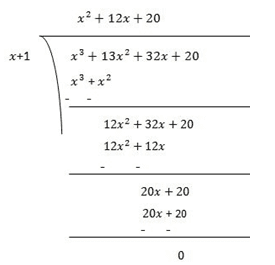Guru

# Factorize:(iii) x3+13×2+32x+20 Q.5(3)

• 0

What is the method for solving the problem of class 9th ncert book of chapter Polynomials chapter of exercise 2.4 of math, give me the best way for solving this question Factorize:(iii) x3+13×2+32x+20

Share

1. Let p(x) = x3+13x2+32x+20

Factors of 20 are ±1, ±2, ±4, ±5, ±10 and ±20

By trial method, we find that

p(-1) = 0

So, (x+1) is factor of p(x)

Now,

p(x)= x3+13x2+32x+20

p(-1) = (−1)3+13(−1)2+32(−1)+20

= −1+13−32+20

= 0

Therefore, (x+1) is the factor of p(x)Now, Dividend = Divisor × Quotient +Remainder

(x+1)(x2+12x+20) = (x+1)(x2+2x+10x+20)

= (x−5)x(x+2)+10(x+2)

= (x−5)(x+2)(x+10)

• 0